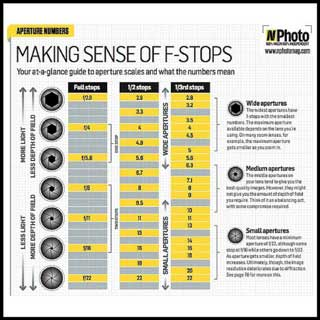What is a STOP?

In photography a stop is the exposure value plus the difference of twice the amount of light to increase the exposure value. ½ the amount of light decreases the exposure value a stop. Fortunately, we can use the camera's built in light meter to figure out a given exposure value.A stop is the exposure value plus the difference of twice the light or ½ the light. For example, if I have a correct exposure with a shutter speed of 1 second and I change the shutter speed to ½ second the I am decreasing the exposure by 1 stop because I am now letting in ½ the light. If I increase from 1 second to 2 seconds I am increasing the exposure value by doubling the time light can hit the camera's sensor, hence an increase of 1 stop. Remember that we normally shoot in fractions of a second so 1/100th of a second to 1/200th of a seconds, which is a shorter amount of time, lets in half the light or decreases the exposure value by a stop. Likewise 1/50th of a second would allow twice the amount of light of 1/100th so 1/50th would increase the exposure value by one stop. 1/25th second would be a two stop increase. The ISO and

Aperture settings, also know as F-Stops work in a similar fashion. If I change my f-stop from f/5 6 to f/4 I will let in twice the amount of light. Conversely a change from 4 to 5.6 cuts the amount of light by half. If I go from 8 to 4 I'll let in 4 times the amount of light which is a 2 stop increase. F-stops can seem confusing because the smaller the f-stop the more light is let in.Finally, the camera's ISO dial either makes the camera's sensor twice as sensitive to light or half as sensitive depending on which direction you are going. ISO 400 is 4 times more sensitive to light than ISO 100 and ISO 200 is twice as sensitive to light as ISO 100. So ISO 400 would be a 2 stop increase if your image was exposed correctly at ISO 100.

If you want to learn the math behind stops, f-stops, exposure values which seems complicated to me, then you may want to watch Exposing Digital Photography series. I've included the link below to the 1st course. So you see, a stop is not the same as an f-stop.